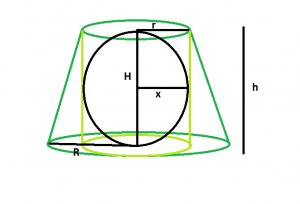# Largest sphere that can be inscribed in a right circular cylinder inscribed in a frustum

Given here is a frustum of height h, top-radius r & base-radius R, which inscribes a right circular cylinder which in turn inscribes a sphere . The task is to find the largest possible volume of this sphere.

Examples:

```Input: r = 5, R = 8, h = 11
Output: 523.333

Input: r = 9, R = 14, h = 20
Output:3052.08
```

## Recommended: Please try your approach on {IDE} first, before moving on to the solution.Approach: Let the height of the cylinder = H, radius of the sphere = x
We know, the height and radius of the cylinder inscribed within the frustum is equal to the height and top-radius of the frustum respectively(Please refer here).So the height of the cylinder = h, radius of the cylinder = r.
Also, radius of the sphere inscribed within a cylinder is equal to radius of the cylinder(Please refer here), so x = r.
So, volume of the sphere, V = 4*π*r^3/3.

Below is the implementation of the above approach:

## C++

 `// C++ Program to find the biggest sphere ` `// that can be inscribed within a right ` `// circular cylinder which in turn is inscribed ` `// within a frustum ` `#include ` `using` `namespace` `std; ` ` `  `// Function to find the biggest sphere ` `float` `sph(``float` `r, ``float` `R, ``float` `h) ` `{ ` ` `  `    ``// the radii and height cannot be negative ` `    ``if` `(r < 0 && R < 0 && h < 0) ` `        ``return` `-1; ` ` `  `    ``// radius of the sphere ` `    ``float` `x = r; ` ` `  `    ``// volume of the sphere ` `    ``float` `V = (4 * 3.14 * ``pow``(r, 3)) / 3; ` ` `  `    ``return` `V; ` `} ` ` `  `// Driver code ` `int` `main() ` `{ ` `    ``float` `r = 5, R = 8, h = 11; ` `    ``cout << sph(r, R, h) << endl; ` ` `  `    ``return` `0; ` `} `

## Java

 `// Java Program to find the biggest sphere ` `// that can be inscribed within a right ` `// circular cylinder which in turn is inscribed ` `// within a frustum ` `import` `java.lang.Math; ` ` `  `class` `gfg ` `{ ` `     `  `// Function to find the biggest sphere ` `static` `float` `sph(``float` `r, ``float` `R, ``float` `h) ` `{ ` ` `  `    ``// the radii and height cannot be negative ` `    ``if` `(r < ``0` `&& R < ``0` `&& h < ``0``) ` `        ``return` `-``1``; ` ` `  `    ``// radius of the sphere ` `    ``float` `x = r; ` ` `  `    ``// volume of the sphere ` `    ``float` `V = (``float``)(``4` `* ``3``.14f * Math.pow(r, ``3``)) / ``3``; ` ` `  `    ``return` `V; ` `} ` ` `  `// Driver code ` `public` `static` `void` `main(String[] args) ` `{ ` `    ``float` `r = ``5``, R = ``8``, h = ``11``; ` `    ``System.out.println(sph(r, R, h)); ` `} ` `} ` ` `  `// This Code is contributed by Code_Mech. `

## Python3

 `# Python3 Program to find the biggest sphere ` `# that can be inscribed within a right ` `# circular cylinder which in turn is inscribed ` `# within a frustum ` `import` `math as mt ` ` `  `# Function to find the biggest sphere ` `def` `sph(r, R, h): ` ` `  `    ``# the radii and height cannot ` `    ``# be negative ` `    ``if` `(r < ``0` `and` `R < ``0` `and` `h < ``0``): ` `        ``return` `-``1` ` `  `    ``# radius of the sphere ` `    ``x ``=` `r ` ` `  `    ``# volume of the sphere ` `    ``V ``=` `(``4` `*` `3.14` `*` `pow``(r, ``3``)) ``/` `3` ` `  `    ``return` `V ` ` `  `# Driver code ` `r, R, h ``=` `5``, ``8``, ``11` `print``(sph(r, R, h)) ` ` `  `# This code is contributed by  ` `# Mohit kumar 29 `

## C#

 `// C# Program to find the biggest sphere ` `// that can be inscribed within a right ` `// circular cylinder which in turn is  ` `// inscribed within a frustum ` `using` `System; ` ` `  `class` `gfg ` `{ ` `     `  `    ``// Function to find the biggest sphere ` `    ``static` `float` `sph(``float` `r, ``float` `R, ``float` `h) ` `    ``{ ` `     `  `        ``// the radii and height  ` `        ``// cannot be negative ` `        ``if` `(r < 0 && R < 0 && h < 0) ` `            ``return` `-1; ` `     `  `        ``// radius of the sphere ` `        ``float` `x = r; ` `     `  `        ``// volume of the sphere ` `        ``float` `V = (``float``)(4 * 3.14f *  ` `                    ``Math.Pow(r, 3)) / 3; ` `     `  `        ``return` `V; ` `    ``} ` `     `  `    ``// Driver code ` `    ``public` `static` `void` `Main() ` `    ``{ ` `        ``float` `r = 5, R = 8, h = 11; ` `        ``Console.WriteLine(sph(r, R, h)); ` `    ``} ` `} ` ` `  `// This code is contributed by Ryuga `

## PHP

 ` `

Output:

```523.333
```

Attention reader! Don’t stop learning now. Get hold of all the important DSA concepts with the DSA Self Paced Course at a student-friendly price and become industry ready.

My Personal Notes arrow_drop_upCheck out this Author's contributed articles.

If you like GeeksforGeeks and would like to contribute, you can also write an article using contribute.geeksforgeeks.org or mail your article to contribute@geeksforgeeks.org. See your article appearing on the GeeksforGeeks main page and help other Geeks.

Please Improve this article if you find anything incorrect by clicking on the "Improve Article" button below.